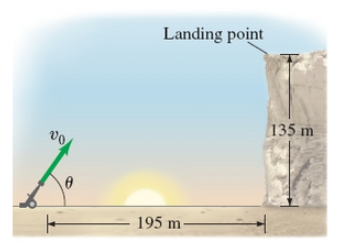# Problem: A projectile is launched from ground level to the top of a cliff which is 195 m away and 135 m high (as shown in the figure ).If the projectile lands on top of the cliff 6.5s after it is fired, find the initial velocity of the projectile ( (a)magnitude and (b)direction ). Neglect air resistance.

###### FREE Expert Solution

In this problem, we'll use vector magnitude:

$\overline{)\mathbf{|}\stackrel{\mathbf{⇀}}{\mathbit{A}}\mathbf{|}{\mathbf{=}}\sqrt{{{\mathbit{A}}_{\mathbit{x}}}^{\mathbf{2}}\mathbf{+}{{\mathbit{A}}_{\mathbit{y}}}^{\mathbf{2}}}}$

89% (327 ratings)###### Problem Details
A projectile is launched from ground level to the top of a cliff which is 195 m away and 135 m high (as shown in the figure ).If the projectile lands on top of the cliff 6.5s after it is fired, find the initial velocity of the projectile ( (a)magnitude and (b)direction ). Neglect air resistance.

Frequently Asked Questions

What scientific concept do you need to know in order to solve this problem?

Our tutors have indicated that to solve this problem you will need to apply the Positive (Upward) Launch concept. You can view video lessons to learn Positive (Upward) Launch. Or if you need more Positive (Upward) Launch practice, you can also practice Positive (Upward) Launch practice problems.

What professor is this problem relevant for?

Based on our data, we think this problem is relevant for Professor Staff's class at OSU.